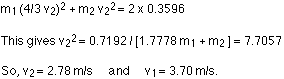## Friday, 31 October 2014

### SOUND QUESTIONS

1. How do sound vibrations of atoms differ from thermal motion?

2. When sound passes from one medium to another where its propagation speed is different, does its frequency or wavelength change? Explain your answer briefly.

3. Six members of a synchronized swim team wear earplugs to protect themselves against water pressure at depths, but they can still hear the music and perform the combinations in the water perfectly. One day, they were asked to leave the pool so the dive team could practice a few dives, and they tried to practice on a mat, but seemed to have a lot more difficulty. Why might this be?

4. A community is concerned about a plan to bring train service to their downtown from the town’s outskirts. The current sound intensity level, even though the rail yard is blocks away, is 70 dB downtown. The mayor assures the public that there will be a difference of only 30 dB in sound in the downtown area. Should the townspeople be concerned? Why?

5. Is the Doppler shift real or just a sensory illusion?

6. Due to efficiency considerations related to its bow wake, the supersonic transport aircraft must maintain a cruising speed that is a constant ratio to the speed of sound (a constant Mach number). If the aircraft flies from warm air into colder air, should it increase or decrease its speed? Explain your answer.

7. When you hear a sonic boom, you often cannot see the plane that made it. Why is that?

8. How does an un amplified guitar produce sounds so much more intense than those of a plucked string held taut by a simple stick?

9. You are given two wind instruments of identical length. One is open at both ends, whereas the other is closed at one end. Which is able to produce the lowest frequency?

10. What is the difference between an overtone and a harmonic? Are all harmonics overtones? Are all overtones harmonics?

11. If audible sound follows a rule of thumb similar to that for ultrasound, in terms of its absorption, would you expect the high or low frequencies from your neighbor’s stereo to penetrate into your house? How does this expectation compare with your experience?

12. Elephants and whales are known to use infra sound to communicate over very large distances. What are the advantages of infra sound for long distance communication?

13. It is more difficult to obtain a high-resolution ultrasound image in the abdominal region of someone who is overweight than for someone who has a slight build. Explain why this statement is accurate.

14. Suppose you read that 210-dB ultrasound is being used to pulverize cancerous tumors. You calculate the intensity in watts per centimeter squared and find it is unreasonably high ( 105 W/cm2). What is a possible explanation?

### FIRST LAW OF THERMODYNAMICS QUESTIONS

1. Describe the photo of the tea kettle at the beginning of this section in terms of heat transfer, work done, and internal energy. How is heat being transferred? What is the work done and what is doing it? How does the kettle maintain its internal energy?

2. The first law of thermodynamics and the conservation of energy, as discussed in Conservation of Energy, are clearly related. How do they differ in the types of energy considered?

3. Heat transfer Q and work done W are always energy in transit, whereas internal energy U is energy stored in a system. Give an example of each type of energy, and state specifically how it is either in transit or resides in a system.

4. How do heat transfer and internal energy differ? In particular, which can be stored as such in a system and which cannot?

5. If you run down some stairs and stop, what happens to your kinetic energy and your initial gravitational potential energy?

6. Give an explanation of how food energy (calories) can be viewed as molecular potential energy (consistent with the atomic and molecular definition of internal energy).

7. Identify the type of energy transferred to your body in each of the following as either internal energy, heat transfer, or doing work: (a) basking in sunlight; (b) eating food; (c) riding an elevator to a higher floor

## Thursday, 30 October 2014

### DENSITY PROBLEMS

1) If you take 1 cm3  of iron ( A small cube) and 1 cm3 of wood, which cube will be lighter (smaller mass ?) ___________
If you measure the mass of 1 cm3 of iron you get 8g. The same volume of oak wood (1cm3) has a mass of 0.7g. We say the density of iron is 8g
cm3  and the density of wood is 0.7g/ cm3     .
Density is the mass of 1 cm3 of matter.
To find the density of a substance, you need to divide the mass (g) by the volume (cm3).
The mass an be in kg and the volume in m3

2) An object has a mass of 100 grams and a volume of 30 cubic centimeters. What is its density?

3) AN object has a mass of 40 000 kilograms and a volume of 5 cubic meters. What is its density>

4) An object has a volume of 3 cubic meters and a density of 6000 kilograms per cubic meter. What is its mass ?

5) Can you explain how a lava lamp works? it has to do with density of water and wax and heat.

## Monday, 27 October 2014

### speed , velocity and displacement problems

1.   A car is traveling at 30. meters per second.  How much distance does it cover in 1 second?
In 2 seconds?      In a minute?

2.  a/   A jet ski moves 280 meters north in 6.3 seconds.   What is its speed?

b/   What is its velocity?

3.   a/  A bicycle moves at 15 m/s east for 2.5 seconds.   What distance does it travel?

b/    What is its displacement?

4.   If a car travels at 35 m/s, how much time does it take to travel 2 km?   (Hint: You MUST change the km to meters before doing the math!)

5.   Two cars, each traveling at 50 mph, are heading in opposite directions.   Compare their speeds and velocities.

6.  See page 1 of the Reference Tables to find the speed of sound in air at STP =  ________ m/s
How far does sound travel in air in 5.0 s?

7.   See page 1 of the Reference Tables to find the speed of light in a vacuum =  ______________ m/s
How much time does it take light to travel 30 cm (30 x 10-2 m), which is about 1 foot?

8.  a/  If car A travels twice as far as car B in the same time, how does vA compare to vB?
What if car A travels three times as far?
Is speed related directly or inversely to distance (if time is constant)?

b/   If car X takes twice as much time as car Y to travel the same distance, how does vX compare to vY?
What if car X takes three times as much time?
Is speed related directly or inversely to time (if distance is constant)?

9.   A car travels 90. meters due north in 15 seconds.   Then the car turns around and travels 40. meters due south for 5.0 seconds.
a/   Remember that average speed = distance / time.    Find the average speed in the first 15 seconds alone.

b/  Now find it for only the last 5.0 seconds.

c/   Now find it for the entire 20. seconds.    (Hint: Use total distance.)

d/  Why does your third answer NOT equal the average of your first two?

e/  Find the average velocity in the first 15 seconds.  Remember that average velocity = displacement/time, so your answer will have a direction.

f/    Now find average velocity during the last 5.0 seconds.

g/     Now over the entire 20. seconds.   (Hint: Use the resultant displacement over the entire 20 s.)

h/   Why is your average velocity (part g/) so different from your average speed (part c/)?

## Friday, 24 October 2014

### FORCE = MASS X ACCELERATION

Directions: On this worksheet, positive horizontal directions are towards the right and positive vertical directions are upwards.
 Question 1  What is the net force acting on a 7-kg mass if it is accelerating at a rate of 5 m/sec2?Question 2  What is the magnitude of the frictional force present if a 7-kg mass being pulled by a horizontal force of 5 newtons towards the right experiences an acceleration of -2.29 m/sec2?3.12 x 10-1 nts 3.06 x 100 nts 2.1 x 101 nts 1.6 x 101 nts

Question 3  A 7-kg mass experiences two vertical forces: 62.6 newtons pulling upward and 68.6 newtons pulling downward. What acceleration does the 7-kg mass undergo?-0.9 m/sec2 6.0 m/sec2 1.0 m/sec2 18.7 m/sec2

Question 4  A 7-kg mass is being pulled towards the right with a rope that has a tension of 35 newtons at an angle of 29 degrees to the x-axis. If the surface is frictionless, what acceleration will the mass develop?2.4 m/sec2 5.0 m/sec2 1.9 m/sec2 4.4 m/sec2

Question 5  A 10-gram bullet travelling at 40 m/sec is fired into a tree. If the bullet is brought to a stop in a distance of 29 centimeters, what average resistive force did the tree exert upon it?-1.38 x 100 nts -2.76 x 101 nts -2.76 x 102 nts -4 x 10-1 nts

### IMPULSE AND WORK

Directions: On this worksheet you will examine the properties of force vs time (impulse) and force vs distance (work) as they apply to two objects of different masses.Question 1  Two masses, 3 kg and 6 kg, initially at rest, are both pushed by equal forces through the same distance across identical frictionless surfaces. Which mass would experience the greater acceleration?
 3 kg 6 kg both masses experience the same acceleration

Question 2  Which mass has the greater final velocity?
 6 kg 3 kg both masses experience the same increase in velocity

Question 3  Which mass gained the greater amount of kinetic energy?
 6 kg 3 kg both masses gain the same amount of kinetic energy

Question 4  Which mass received the greater impulse?
 both masses require the same amount of time to reach the end 3 kg 6 kg

Question 5  If each mass was pushed by a 30-N force through a distance of 78 meters, what is the ratio of the change in momentum of the 3-kg mass to that of the 6-kg mass?
 1.41:1 0.71:1 1:1 0.50:1

Question 6  What is the final velocity of the 3-kg mass?
 5.0 m/sec 39.5 m/sec 27.9 m/sec 10.0 m/sec

Question 7  How much time was required for the 6-kg mass to travel the entire 78 meters?
 1.97 sec 2.79 sec 5.59 sec 3.95 sec

Question 8  Which formula correctly relates an object's kinetic energy and its momentum?
 KE = ½(p2/m) KE = 2m/p2 KE = ½(p2/v) KE = 2v/p2

Question 9  Regardless of which mass is being examined, which graph will always has the greater area?
 both are always equal Force vs Distance neither is always larger Force vs Time

## Thursday, 23 October 2014

### MOMENTUM, IMPULSE QUESTIONS

1.  A 1.2-kg object moving with a speed of 8.0 m/s collides perpendicularly with a wall and emerges with a speed of 6.0 m/s in the opposite direction. If the object is in contact with the wall for 2.0 ms, what is the magnitude of the average force on the object by the wall?
A) 9.8 Kilo Newton
B) 8.4 Kilo Newton
C) 7.7 Kilo Newton
D) 9.1 Kilo Newton
E) 1.2 Kilo Newton

2.  The speed of a 2.0-kg object changes from 30 m/s to 40 m/s during a 5.0-s time interval. During this same time interval, the velocity of the object changes its direction by 90°. What is the magnitude of the average total force acting on the object during this time interval?
A) 30 N
B) 20 N
C) 40 N
D) 50 N
E) 6.0 N

3.  A 2.4-kg ball falling vertically hits the floor with a speed of 2.5 m/s and rebounds with a speed of 1.5 m/s. What is the magnitude of the impulse exerted on the ball by the floor?
A) 9.6 N s
B) 2.4 N s
C) 6.4 N s
D) 1.6 N s
E) 1.0 N s

4.  A 1.6-kg ball is attached to the end of a 0.40-m string to form a pendulum. This pendulum is released from rest with the string horizontal. At the lowest point of its swing, when it is moving horizontally, the ball collides with a 0.80-kg block initially at rest on a horizontal frictionless surface. The speed of the block just after the collision is 3.0 m/s. What is the speed of the ball just after the collision?
A) 1.7 m/s
B) 1.1 m/s
C)1.5 m/s
D) 1.3 m/s
E)2.1 m/s

5.  A 2.0-kg object moving with a velocity of 5.0 m/s in the positive x direction strikes and sticks to a 3.0-kg object moving with a speed of 2.0 m/s in the same direction. How much kinetic energy is lost in this collision?
A) 2.4 J
B) 9.6 J
C) 5.4 J
D) 0.6 J
E) 6.0 J

6.  A 12-g bullet moving horizontally strikes and remains in a 3.0-kg block initially at rest on the edge of a table. The block, which is initially 80 cm above the floor, strikes the floor a horizontal distance of 120 cm from its initial position. What was the initial speed of the bullet?
A) 0.68 km/s
B) 0.75 km/s
C) 0.81 km/s
D) 0.87 km/s
E) 0.41 km/s

7.  A 2.0-kg object moving 5.0 m/s collides with and sticks to an 8.0-kg object initially at rest. Determine the kinetic energy lost by the system as a result of this collision.
A) 20 J
B) 15 J
C) 30 J
D) 25 J
E) 5.0 J

8.  A 3.0-kg mass sliding on a frictionless surface has a velocity of 5.0 m/s east when it undergoes a one-dimensional inelastic collision with a 2.0-kg mass that has an initial velocity of 2.0 m/s west. After the collision the 3.0-kg mass has a velocity of 1.0 m/s east. How much kinetic energy does the two-mass system lose during the collision?
A) 22 J
B) 24 J
C) 26 J
D) 20 J
E) 28 J

### WORK ,ENERGY,POWER QUESTIONS AP

AP Conservation of Energy and Power

1.    An electrical motor lifts a 575 N box 20 m straight up by a rope in 10 s. What power is developed by the motor? (1.1510W)

2.     A block slides down a frictionless inclined plane of height h = 1 m, making angle θ with the horizontal. At the bottom of the plane, the block continues to move on a flat surface with a coefficient of friction μ = 0.30. How far does the mass move on the flat surface? (3.3m)

3.     A cyclist approaches the bottom of a hill at a speed of 11 m/s. The hill is 6 m high. Ignoring friction, how fast is the cyclist moving at the top of the hill? Assume that he doesn’t peddle and ignore air resistance. (1.81m/s).

4.    A 100 kg mass traveling with a velocity of 15 m/s on a horizontal surface strikes a spring with a spring constant k = 5 N/m.
a. Find the compression of the spring required to stop the mass if the surface is frictionless.(67.1m)
b. Find the compression of the spring if the surface is rough (0.4kμ=0). (x = 25 m)

5.         An amusement park roller coaster car has a mass of 250 kg. During the ride, it is towed to the top of a 30 m hill, where it is released from rest and allowed to roll. The car plunges down the hill, then up a 10 m hill and through a loop with a radius of 10 m. Assume that the tracks are frictionless. (Use g = 10 m/s2.)

a. What is the Potential Energy of the car at the top of the 30 m hill?(75000 J)
b. What are the Kinetic Energy and the speed of the car at the bottom of the 30 m hill? (75000 J) (24 m/s)
c. What are the Kinetic Energy and the speed of the car at the top of the 10 m hill? (50000J) (20m/s)
d. If the hill makes an angle of 60o with the horizontal and the car takes 15 seconds to be towed up the hill, determine the length of the hill, the velocity of the car, the force required to tow the car up the hill, and the power of the motor pulling the car up the hill.(5KW)

## Monday, 20 October 2014

### MAGNETIC FORCE QUESTIONS

1. Suppose that there are 8.5x1028 conduction electrons per m³ of copper. A piece of copper wire has a cross-sectional area of 1 mm². A current of 0.1 A flows through it. How many electrons flow past a point P on the wire in 1 s?
2. Find the volume of copper that contains this number of electrons. Find the length of the copper wire that contains has the same volume.
3. Since the conduction electrons in this length of the wire flows past point P in 1 s, find the average velocity of these electrons.
4. Find the force on this length of wire if a magnetic flux density of 0.1 T is at right angle to it.
5. Using this force, find the average force on one conduction electron.
6. Find this force again using F = BQv.

### WAVES AND INTERFERENCE QUESTIONS

1. Two narrow rods P and Q are held vertically at 10 cm apart. They move up and down together 4 times per second into the water in a ripple tank. Suppose that a peak of a ripple travels at 20 cm/s. Find the wavelength of the ripples.
2. The midpoint between the rods is O. Suppose that a point R is 20 cm from P and 22.5 cm from Q. Sketch a diagram to show the positions of O, P, Q and R. Label the distances.
3. Calculate the number of wavelengths along the line PR and the line QR. Describe and explain what happens at R.
4. Draw a line through R that is parallel to PQ. Mark the point that is equidistant from P and Q. Label it S. Describe and explain what happens at S.
5. Describe and explain what happens if you start from S and move along the line parallel to PQ to R, and carry on moving beyond R.
6. Sketch a graph of the amplitude of the waves against distance from S along this line.
7. Describe the wave along the straight line joining the two rods if rod P is not there. Describe the wave if rod Q is not there. Describe and explain the wave if both rods are present.

### GRAVITATION QUESTIONS

1. Suppose that a satellite always stays directly above the same spot on Earth’s equator. Find the period of revolution of the satellite in SI units.
2. Find its angular velocity.
3. Using GMm/r² and mω²r, write down an equation relating the gravitational and centripetal forces on the satellite. Explain.
4. Rearrange this equation to make r the subject.
5. Find r. Find the height of the satellite above the equation. Mass of Earth is 5.97x1024 kg.
6. How many times is this distance bigger than the 6380 km radius of the Earth.
7. What is the name of this type of satellite?

### PHOTOELECTRIC EFFECT QUESTIONS

1. There are two metal plates A and B in a glass tube. The air in the tube has been mostly pumped out. When a 470 nm light shines on A, a voltage is measured across AB. What is the colour of this light?2. Why does a voltage appear across AB? If we think of this as a cell, which plate is the positive pole?
3. Find the frequency of the light. Find the energy of each of its photons.
4. It takes at least 2.28 eV to remove an electron from metal A. What is the name of this energy?
5. An electron absorbs the energy of one of the photons and jumps out of the metal plate. What is the range of possible energies of this electron?
6. Why do the emitted electrons not all have the same energy?
7. If A and B are connected by a piece of wire, a current flows. I want to connect a battery in the reverse direction to A and B to stop this current. What voltage must I use?
8. What do you think the voltage in (ii) above might be? Why?

## Friday, 10 October 2014

### VELOCITY PROBLEMS

1.The fastest fish, the sailfish, can swim 1.2 × 102 km/h. Suppose you have
a friend who lives on an island 16 km away from the shore. If you send
a message using a sailfish as a messenger, how long will it take for the
message to reach your friend?
2. The Sears Tower in Chicago is 443 m tall. Joe wants to set the world’s
stair climbing record and runs all the way to the roof of the tower. If Joe’s
average upward speed is 0.60 m/s, how long will it take Joe to climb from
street level to the roof of the Sears Tower?
3. An ostrich can run at speeds of up to 72 km/h. How long will it take an
ostrich to run 1.5 km at this top speed?
4. A cheetah is known to be the fastest mammal on Earth, at least for short
runs. Cheetahs have been observed running a distance of 5.50 × 102 m
with an average speed of 1.00 × 102 km/h.
a. How long would it take a cheetah to cover this distance at this speed?
b. Suppose the average speed of the cheetah were just 85.0 km/h.
What distance would the cheetah cover during the same time interval
calculated in (a)?

4. A pronghorn antelope has been observed to run with a top speed of
97 km/h. Suppose an antelope runs 1.5 km with an average speed of
85 km/h, and then runs 0.80 km with an average speed of 67 km/h.
a. How long will it take the antelope to run the entire 2.3 km?
b. What is the antelope’s average speed during this time?
5. Jupiter, the largest planet in the solar system, has an equatorial radius of
about 7.1 × 104 km (more than 10 times that of Earth). Its period of rotation,
however, is only 9 h, 50 min. That means that every point on
Jupiter’s equator “goes around the planet” in that interval of time. Calculate
the average speed (in m/s) of an equatorial point during one period
of Jupiter’s rotation. Is the average velocity different from the average
speed in this case?
6. The peregrine falcon is the fastest of flying birds (and, as a matter of fact,
is the fastest living creature). A falcon can fly 1.73 km downward in 25 s.
What is the average velocity of a peregrine falcon?
7. The black mamba is one of the world’s most poisonous snakes, and with
a maximum speed of 18.0 km/h, it is also the fastest. Suppose a mamba
waiting in a hide-out sees prey and begins slithering toward it with a
velocity of +18.0 km/h. After 2.50 s, the mamba realizes that its prey can
move faster than it can. The snake then turns around and slowly returns
to its hide-out in 12.0 s. Calculate
a. the mamba’s average velocity during its return to the hideout.
b. the mamba’s average velocity for the complete trip.
c. the mamba’s average speed for the complete trip.
8. In the Netherlands, there is an annual ice-skating race called the “Tour of
the Eleven Towns.” The total distance of the course is 2.00 × 102 km, and
the record time for covering it is 5 h, 40 min, 37 s.
a. Calculate the average speed of the record race.
b. If the first half of the distance is covered by a skater moving with
a speed of 1.05v, where v is the average speed found in (a), how
long will it take to skate the first half? Express your answer in hours
and minutes.

### MEASUREMENT PROBLEMS

1.In Hindu chronology, the longest time measure is a para. One para equals311 040 000 000 000 years.
Calculate this value in megahours and in nanoseconds.Write your answers in scientific notation.

2. One light-year is the distance light travels in one year. This distance is equal to 9.461 × 1015 m. After the sun, the star nearest to Earth is Alpha Centauri, which is about 4.35 light-years from Earth. Express this distance in
a. megameters.
b. picometers.

3. It is estimated that the sun will exhaust all of its energy in about ten
billion years. By that time, it will have radiated about 1.2 × 1044 J (joules)
of energy. Express this amount of energy in
a. kilojoules.
b. nanojoules.
4. The smallest living organism discovered so far is called a mycoplasm. Its
mass is estimated as 1.0 × 10–16 g. Express this mass in
a. petagrams.
b. femtograms.
c. attograms.
5. The “extreme” prefixes that are officially recognized are yocto, which indicates
a fraction equal to 10–24, and yotta, which indicates a factor equal
to 1024. The maximum distance from Earth to the sun is 152 100 000 km.
Using scientific notation, express this distance in
a. yoctometers (ym).
b. yottameters (Ym).
6. In 1993, the total production of nuclear energy in the world was
2.1 × 1015 watt-hours, where a watt is equal to one joule (J) per second.
Express this number in
a. joules.
b. gigajoules.
7. In Einstein’s special theory of relativity, mass and energy are equivalent.
An expression of this equivalence can be made in terms of electron volts
(units of energy) and kilograms, with one electron volt (eV) being equal
to 1.78 × 10–36 kg. Using this ratio, express the mass of the heaviest
mammal on earth, the blue whale, which has an average mass of
1.90 × 105 kg, in
a. mega electron volts.
b. tera electron volts.
8. The most massive star yet discovered in our galaxy is one of the stars
in the Carina Nebula, which can be seen from Earth’s Southern
Hemisphere and from the tropical latitudes of the Northern Hemisphere.
The star, designated as Eta Carinae, is believed to be 200 times as massive
as the sun, which has a mass of nearly 2 × 1030 kg. Find the mass of Eta
Carinae in
a. milligrams.
b. exagrams.
9. The Pacific Ocean has a surface area of about 166 241 700 km2 and an
average depth of 3940 m. Estimate the volume of the Pacific Ocean in
a. cubic centimeters.
b. cubic millimeters.

## Sunday, 5 October 2014

### Relative velocities

Let's say you're standing on an interstate freeway that runs north and south. You see a truck heading north at 60 km/h, and a car heading south at 70 km/h. All three of you (you, the car driver, and the truck driver) agree on these points:
• the truck is traveling at 60 km/h north relative to you, and 130 km/h north relative to the car.
• the car is traveling at 70 km/h south relative to you, and 130 km/h south relative to the truck.
• you are traveling at 60 km/h south relative to the truck, and 70 km/h north relative to the car.
Two observers always agree on their relative velocity. The simple addition we used to get the velocity of the truck relative to the car can not be applied to a relativistic situation, however.Let's say you now stand on an intergalactic freeway. You see a truck heading in one direction at 0.6c, and a car heading in the opposite direction at 0.7c. What is the velocity of the truck relative to the car? It is not 1.3c, because nothing can travel faster than c. The relative velocity can be found using this equation: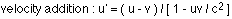In this case, u is the velocity of the car relative to you, v is the velocity of the truck relative to you, and u' is the velocity of the car relative to the truck. Taking the direction the car is traveling to be the positive direction: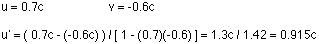So, now everyone involved agrees on this:
• the truck is traveling at 0.6c relative to you, and 0.915c relative to the car.
• the car is traveling at 0.7c relative to you, and 0.915c relative to the truck.
• you are traveling at 0.6c relative to the truck, and 0.7c relative to the car.
The relativistic equation for velocity addition shown above can also be used for non-relativistic velocities. We're more used to adding velocities like this : u' = u - v. This is exactly what the relativistic equation reduces to for velocities much less than the speed of light. The relativistic equation applies to any situation; the one we're used to is a special case that applies only for small velocities.

### EXPLANATION ON MICROSCOPE BY USING RAY DIAGRAM AND EXAMPLE

A basic microscope is made up of two converging lenses. One reason for using two lenses rather than just one is that it's easier to get higher magnification. If you want an overall magnification of 35, for instance, you can use one lens to magnify by a factor of 5, and the second by a factor of 7. This is generally easier to do than to get magnification by a factor of 35 out of a single lens.
A microscope arrangement is shown below, along with the ray diagram showing how the first lens creates a real image. This image is the object for the second lens, and the image created by the second lens is the one you'd see when you looked through the microscope.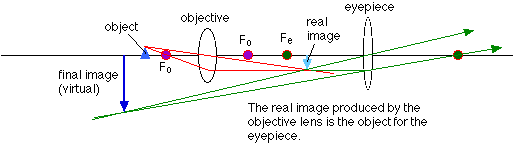Note that the final image is virtual, and is inverted compared to the original object. This is true for many types of microscopes and telescopes, that the image produced is inverted compared to the object.

### An example using the microscope

Let's use the ray diagram for the microscope and work out a numerical example. The parameters we need to specify are: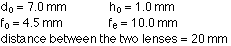To work out the image distance for the image formed by the objective lens, use the lens equation, rearranged to: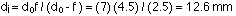The magnification of the image in the objective lens is: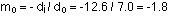So the height of the image is -1.8 x 1.0 = -1.8 mm.
This image is the object for the second lens, and the object distance has to be calculated:The image, virtual in this case, is located at a distance of:The magnification for the eyepiece is: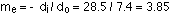So the height of the final image is -1.8 mm x 3.85 = -6.9 mm.
The overall magnification of the two lens system is: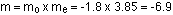This is equal to the final height divided by the height of the object, as it should be. Note that, applying the sign conventions, the final image is virtual, and inverted compared to the object. This is consistent with the ray diagram.

### EXAMPLES TO DETERMINE THE IMAGE DISTANCE AND MAGNIFICATION

EXAMPLE1 FOR CONCAVE MIRROR
A Star Wars action figure, 8.0 cm tall, is placed 23.0 cm in front of a concave mirror with a focal length of 10.0 cm. Where is the image? How tall is the image? What are the characteristics of the image?
The first step is to draw the ray diagram, which should tell you that the image is real, inverted, smaller than the object, and between the focal point and the center of curvature. The location of the image can be found from the mirror equation: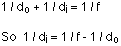which can be rearranged to: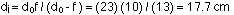The image distance is positive, meaning that it is on the same side of the mirror as the object. This agrees with the ray diagram. Note that we don't need to worry about converting distances to meters; just make sure everything has the same units, and whatever unit goes into the equation is what comes out.
Calculating the magnification gives: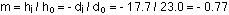Solving for the image height gives:The negative sign for the magnification, and the image height, tells us that the image is inverted compared to the object.
To summarize, the image is real, inverted, 6.2 cm high, and 17.7 cm in front of the mirror.

### The same Star Wars action figure, 8.0 cm tall, is placed 6.0 cm in front of a convex mirror with a focal length of -12.0 cm. Where is the image in this case, and what are the image characteristics?

Again, the first step is to draw a ray diagram. This should tell you that the image is located behind the mirror; that it is an upright, virtual image; that it is a little smaller than the object; and that the image is between the mirror and the focal point.
The second step is to confirm all those observations. The mirror equation, rearranged as in the first example, gives: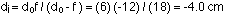Solving for the magnification gives: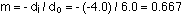This gives an image height of 0.667 x 8 = 5.3 cm.
All of these results are consistent with the conclusions drawn from the ray diagram. The image is 5.3 cm high, virtual, upright compared to the object, and 4.0 cm behind the mirror.

## Friday, 3 October 2014

### KIRCHOFF'S RULE PROBLEM EXPLANATION TO DETERMINE UNKNOWN CURRENTS

Consider the circuit below: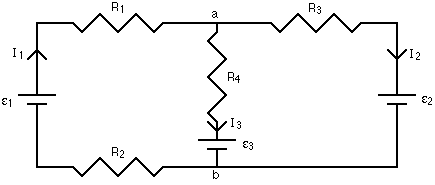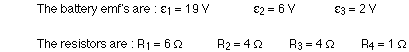Step 1 of the branch current method has already been done. The currents have been labeled in each branch of the circuit, and the directions are shown with arrows. Again, you don't have to be sure of these directions at this point. Simply choose directions, and if any of the currents come out to have negative signs, all it means is that the direction of that current is opposite to the way you've shown on your diagram.
Applying step 2 of the branch current method means looking at the junctions, and writing down a current equation. At junction a, the total current coming in to the junction equals the total current flowing away. This gives:
at junction a : I1 = I2 + I3
If we applied the junction rule at junction b, we'd get the same equation. So, applying the junction rule at one of the junctions is all we need to do. In some cases you will need to get equations from more than one junction, but you'll never need to get an equation for every junction.
There are three unknowns, the three currents, so we need to have three equations. One came from the junction rule; the other two come from going to step 3 and applying the loop rule. There are three loops to use in this circuit: the inside loop on the left, the inside loop on the right, and the loop that goes all the way around the outside. We just need to write down loop equations until each branch has been used at least once, though, so using any two of the three loops in this case is sufficient.
When applying the loop equation, the first step is to choose a starting point on one loop. Then walk around the loop, in either direction, and write down the change in potential when you go through a battery or resistor. When the potential increases, the change is positive; when the potential decreases, the change is negative. When you get back to your starting point, add up all the potential changes and set this sum equal to zero, because the net change should be zero when you get back to where you started.
When you pass through a battery from minus to plus, that's a positive change in potential, equal to the emf of the battery. If you go through from plus to minus, the change in potential is equal to minus the emf of the battery.
Current flows from high to low potential through a resistor. If you pass through a resistor in the same direction as the current, the potential, given by IR, will decrease, so it will have a minus sign. If you go through a resistor opposite to the direction of the current, you're going from lower to higher potential, and the IR change in potential has a plus sign.
Keeping all this in mind, let's write down the loop equation for the inside loop on the left side. Picking a starting point as the bottom left corner, and moving clockwise around the loop gives: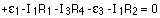Make sure you match the current to the resistor; there is one current for each branch, and a loop has at least two branches in it.
The inner loop on the right side can be used to get the second loop equation. Starting in the bottom right corner and going counter-clockwise gives: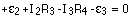Plugging in the values for the resistances and battery emf's gives, for the three equations: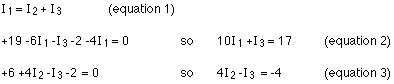The simplest way to solve this is to look at which variable shows up in both loop equations (equations 2 and 3), solve for that variable in equation 1, and substitute it in in equations 2 and 3.
Rearranging equation 1 gives:Substituting this into equation 2 gives: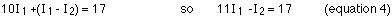Making the same substitution into equation 3 gives: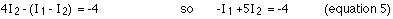This set of two equations in two unknowns can be reduced to one equation in one unknown by multiplying equation 4 by 5 (the number 5, not equation 5!) and adding the result to equation 5.Substituting this into equation 5 gives:
I2 = ( -4 + 1.5 ) / 5 = -0.5 A
The negative sign means that the current is 0.5 A in the direction opposite to that shown on the diagram. Solving for the current in the middle branch from equation 1 gives:
I3 = 1.5 - (-0.5) = 2.0 A
An excellent way to check your answer is to go back and label the voltage at each point in the circuit. If everything is consistent, your answer is fine. To label the voltage, the simplest thing to do is choose one point to be zero volts. It's just the difference in potential between points that matters, so you can define one point to be whatever potential you think is convenient, and use that as your reference point. My habit is to set the negative side of one of the batteries to zero volts, and measure everything else with respect to that.In this example circuit, when the potential at all the points is labeled, everything is consistent. What this means is that when you go from junction b to junction a by any route, and figure out what the potential at a is, you get the same answer for each route. If you got different answers, that would be a big hint that you did something wrong in solving for the currents. Note also that you have to account for any of the currents coming out to be negative, and going the opposite way from what you had originally drawn.
One final note: you can use this method of circuit analysis to solve for more things than just the current. If one or more of the currents was known (maybe the circuit has an ammeter or two, measuring the current magnitude and direction in one or two branches) then an unknown battery emf or an unknown resistance could be found instead.

### SERIES AND PARALLEL COMBINATION OF RESISTORS

EXAMPLE 1
Equivalent resistance of resistors in series : R = R1 + R2 + R3 + ...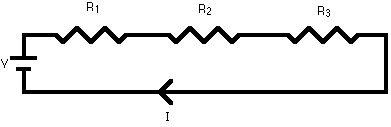A series circuit is shown in the diagram above. The current flows through each resistor in turn. If the values of the three resistors are: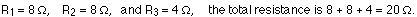With a 10 V battery, by V = I R the total current in the circuit is:
I = V / R = 10 / 20 = 0.5 A. The current through each resistor would be 0.5 A.

EXAMPLE 2

Equivalent resistance of resistors in parallel: 1 / R = 1 / R1 + 1 / R2 + 1 / R3 +...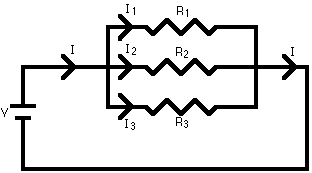A parallel circuit is shown in the diagram above. In this case the current supplied by the battery splits up, and the amount going through each resistor depends on the resistance. If the values of the three resistors are:With a 10 V battery, by V = I R the total current in the circuit is: I = V / R = 10 / 2 = 5 A.
The individual currents can also be found using I = V / R. The voltage across each resistor is 10 V, so:
I1 = 10 / 8 = 1.25 A
I2 = 10 / 8 = 1.25 A
I3=10 / 4 = 2.5 A
Note that the currents add together to 5A, the total current

### RESISTANCE AND COST OF ELECTRICAL ENERGY PROBLEMS

1)A copper wire has a length of 160 m and a diameter of 1.00 mm. If the wire is connected to a 1.5-volt battery, how much current flows through the wire?
The current can be found from Ohm's Law, V = IR. The V is the battery voltage, so if R can be determined then the current can be calculated. The first step, then, is to find the resistance of the wire: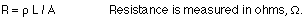L is the length, 1.60 m. The resistivity can be found from the table on page 535 in the textbook.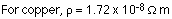The area is the cross-sectional area of the wire. This can be calculated using: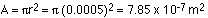The resistance of the wire is then: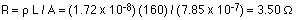The current can now be found from Ohm's Law:
I = V / R = 1.5 / 3.5 = 0.428 A
2)One kW-h typically costs about 10 cents, which is really quite cheap. It does add up, though. The following equation gives the total cost of operating something electrical:
Cost = (Power rating in kW) x (number of hours it's running) x (cost per kW-h)
An example...if a 100 W light bulb is on for two hours each day, and energy costs \$0.10 per kW-h, how much does it cost to run the bulb for a month?
Cost = 0.1 kW x 60 hours x \$0.1/kW-h = \$0.6, or 60 cents.
Try this at home - figure out the monthly cost of using a particular appliance you use every day. Possibilities include hair dryers, microwaves, TV's, etc. The power rating of an appliance like a TV is usually written on the back, and if it doesn't give the power it should give the current. Anything you plug into a wall socket runs at 120 V, so if you know that and the current you can figure out how much power it uses.
The cost for power that comes from a wall socket is relatively cheap. On the other hand, the cost of battery power is much higher. \$100 per kW-h, a thousand times more than what it costs for AC power from the wall socket, is a typical value.

### Two positively-charged balls - FINDING VELOCITY CONSERVATION OF ENERGY

Two positively-charged balls are tied together by a string. One ball has a mass of 30 g and a charge of 1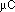; the other has a mass of 40 g and a charge of 2. The distance between them is 5 cm. The ball with the smaller charge has a mass of 30 g; the other ball has a mass of 40 g. Initially they are at rest, but when the string is cut they move apart. When they are a long way away from each other, how fast are they going?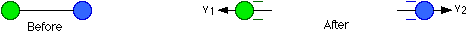Let's start by looking at energy. No external forces act on this system of two charges, so the energy must be conserved. To start with all the energy is potential energy; this will be converted into kinetic energy.
Energy at the start : KE = 0
PE = k q Q / r = (8.99 x 109) (1 x 10-6) (2 x 10-6) / 0.05 = 0.3596 J
When the balls are very far apart, the r in the equation for potential energy will be large, making the potential energy negligibly small.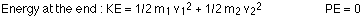Energy is conserved, so the kinetic energy at the end is equal to the potential energy at the start: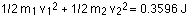The masses are known, but the two velocities are not. To solve for the velocities, we need another relationship between them. Because no external forces act on the system, momentum will also be conserved. Before the string is cut, the momentum is zero, so the momentum has to be zero all the way along. The momentum of one ball must be equal and opposite to the momentum of the other, so: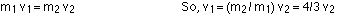Plugging this into the energy equation gives: